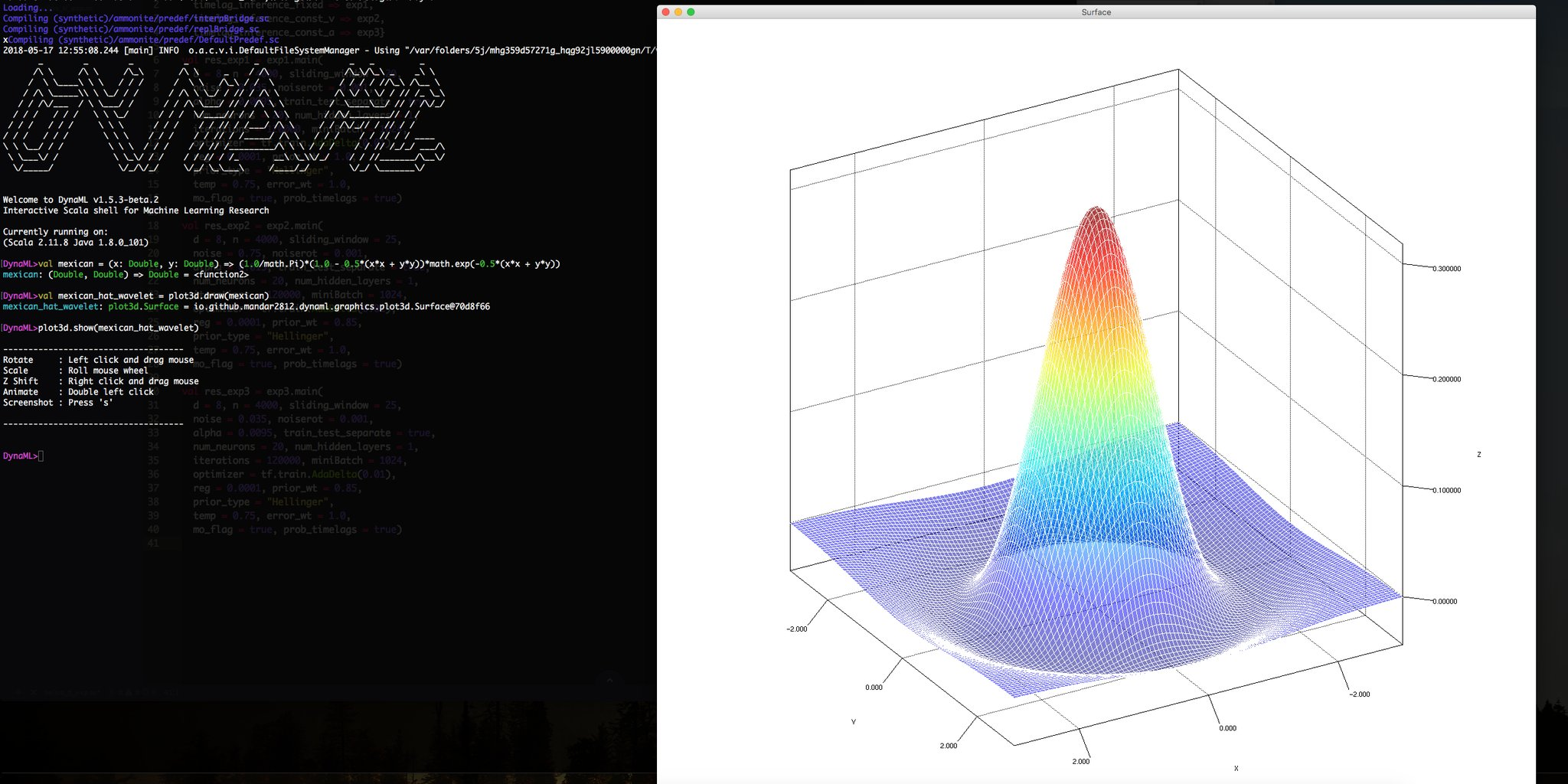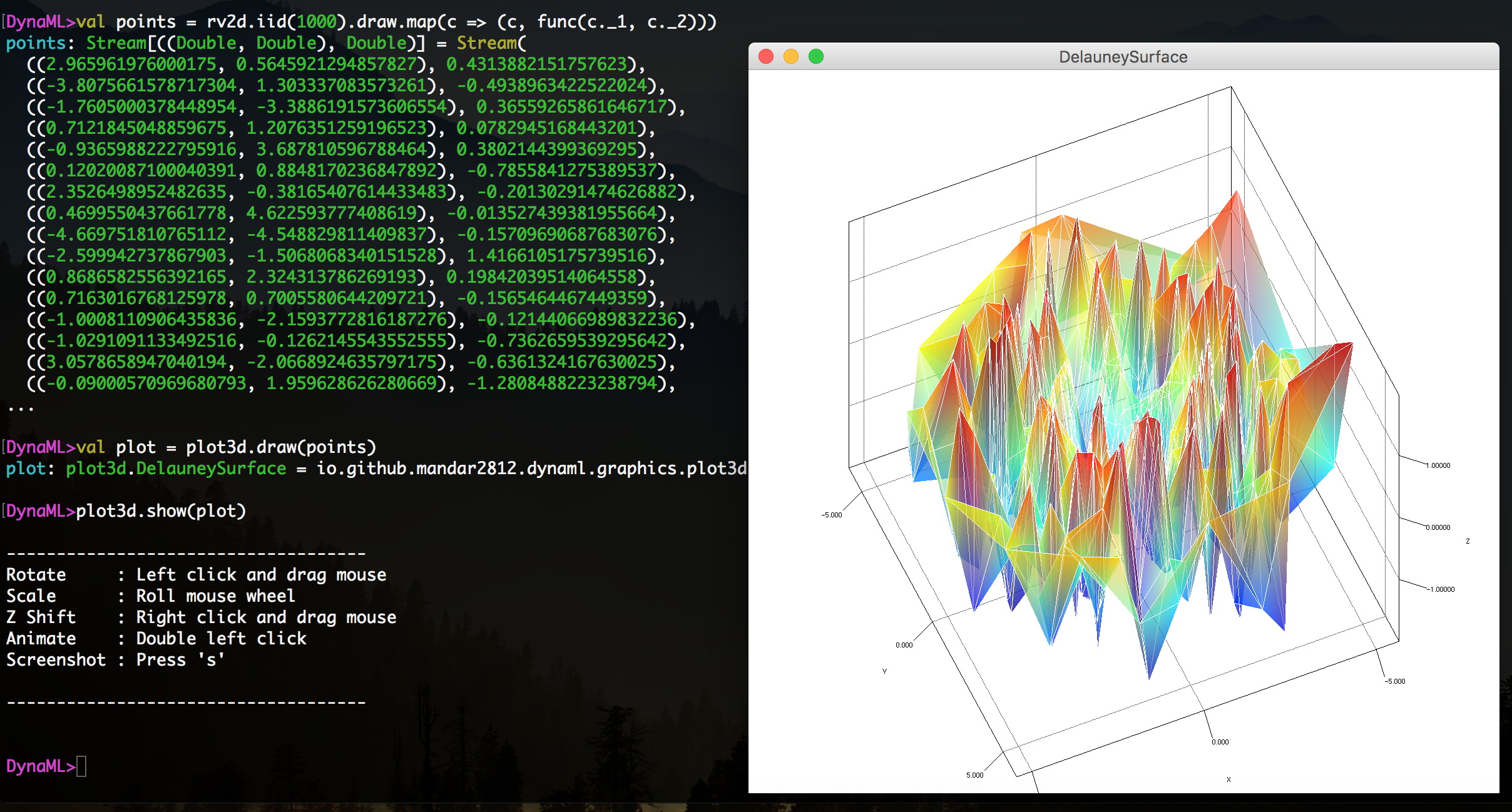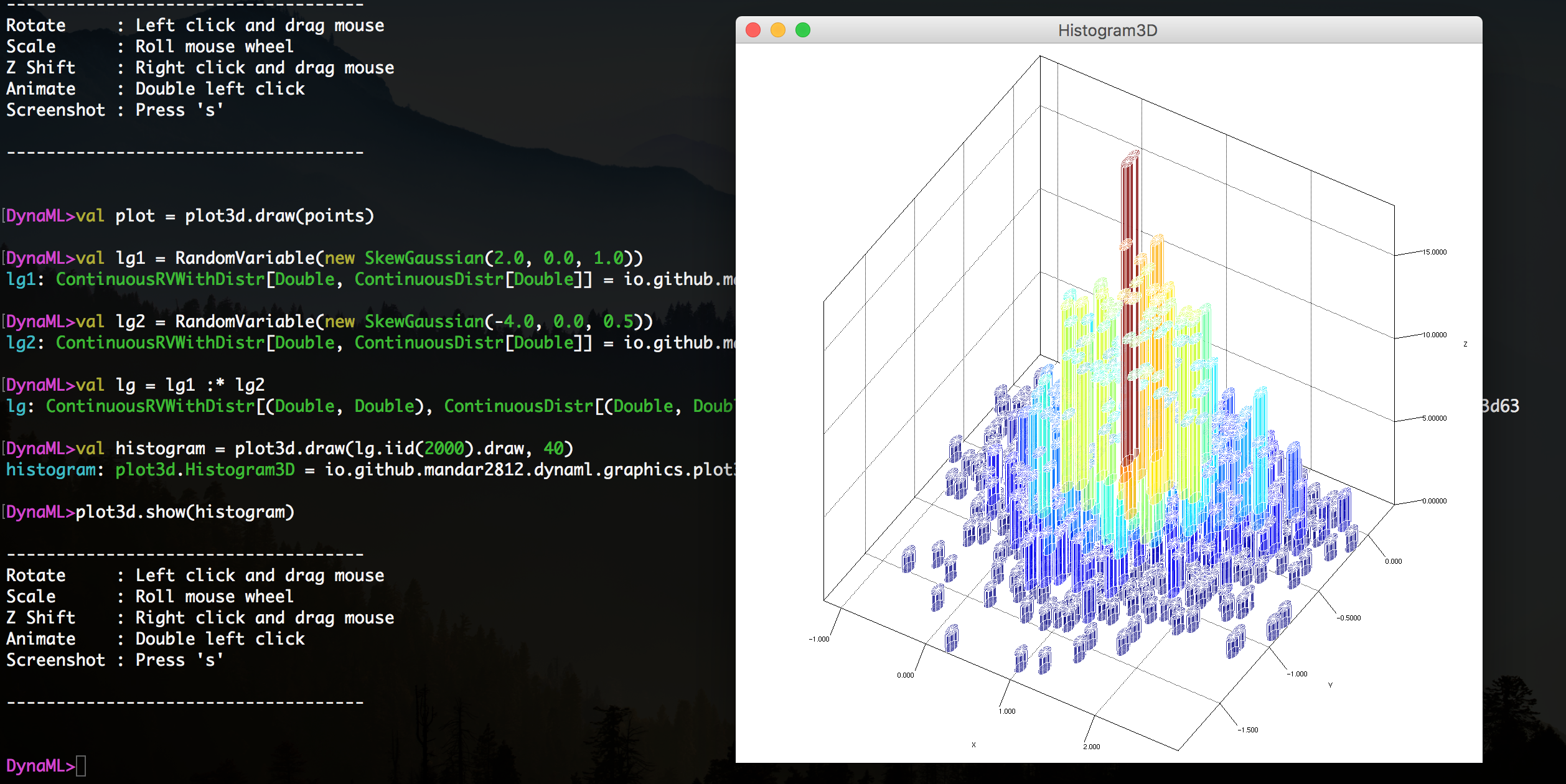# Graphics

Summary

The dynaml.graphics package is a new addition to the API since the v1.5.3 release. It aims to provide more unified access point to producing visualizations.

## 3D Plots¶

Support for 3d visualisations is provided by the dynaml.graphics.plot3d, under the hood the plot3d object calls the Jzy3d library to produce interactive 3d plots.

Producing 3d charts involves similar procedure for each chart kind, use plot3d.draw() to generate a chart object, and use plot3d.show() to display it in a GUI.

### Surface Plots¶

The most common usage of plot3d is to visualise 3 dimensional surfaces, this can be done in two ways.

#### From defined functions¶

If the user can express the surface as a function of two arguments.

import io.github.mandar2812.dynaml.graphics._

val mexican_hat =
(x: Double, y: Double) =>
(1.0/math.Pi)*(1.0 - 0.5*(x*x + y*y))*math.exp(-0.5*(x*x + y*y))

val mexican_hat_chart = plot3d.draw(mexican_hat)

plot3d.show(mexican_hat_chart)#### From a set of points¶

If the surface is not determined completely, but only sampled over a discrete set of points, it is still possible to visualise an approximation to the surface, using Delauney triangulation.

import io.github.mandar2812.dynaml.graphics._
import io.github.mandar2812.dynaml.probability._

//A function generating the surface need not be known,
val func = (x: Double, y: Double) =>
math.sin(x*y + y*x) - math.cos(y*y*x - x*x*y)
//Sample the 2d plane using a gaussian distribution
val rv2d = GaussianRV(0.0, 2.0) :* GaussianRV(0.0, 2.0)
//Generate some random points and their z values
val points = rv2d.iid(1000).draw.map(c => (c, func(c._1, c._2)))

val plot = plot3d.draw(points)
plot3d.show(plot)### Histograms¶

The plot3d object also allows the user to visualise 3d histograms from collections of points on the 2d plane, expressed as a collection of (Double, Double).

import io.github.mandar2812.dynaml.graphics._
import io.github.mandar2812.dynaml.probability._
import io.github.mandar2812.dynaml.probability.distributions._

//Create two different random variables to
//sample x and y coordinates.
val rv_x = RandomVariable(new SkewGaussian(2.0, 0.0, 1.0))
val rv_y = RandomVariable(new SkewGaussian(-4.0, 0.0, 0.5))
//Sample a point on the 2d plane
val rv  = rv_x :* rv_y

val histogram = plot3d.draw(rv.iid(2000).draw, 40)
plot3d.show(histogram)